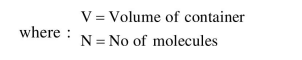# An ideal gas in a closed container is slowly heated. As its temperature increases, which of the following statements are true ?

Question:

An ideal gas in a closed container is slowly heated. As its temperature increases, which of the following statements are true ?

(A) the mean free path of the molecules decreases.

(B) the mean collision time between the molecules decreases.

(C) the mean free path remains unchanged.

(D) the mean collision time remains unchanged.

1. (C) and (D)

2. (A) and (B)

3. (A) and (D)

4. (B) and (C)

Correct Option: , 4

Solution:

The mean free path of molecules of an ideal gas is given as:

$\lambda=\frac{\mathrm{V}}{\sqrt{2} \pi \mathrm{d}^{2} \mathrm{~N}}$Hence with increasing temp since volume of container does not change (closed container), so mean free path is unchanged.

Average collision time

$=\frac{\text { mean free path }}{\mathrm{V}_{\mathrm{av}}}=\frac{\lambda}{\text { (avg speed of molecules) }}$

$\because$ avg speed $\alpha \sqrt{\mathrm{T}}$

$\therefore$ Avg coll. time $\alpha \frac{1}{\sqrt{\mathrm{T}}}$

Hence with increase in temperature the average collision time decreases.